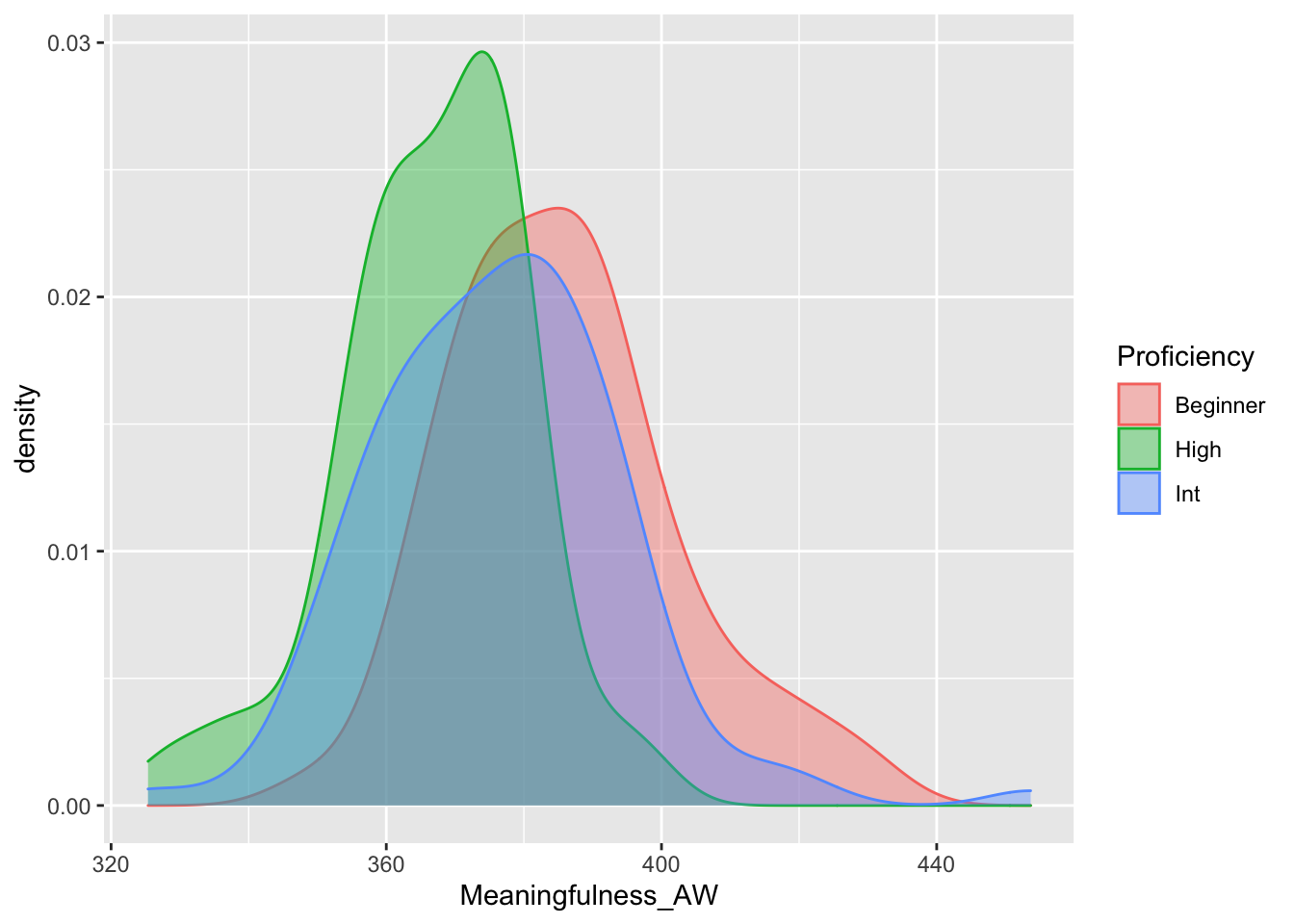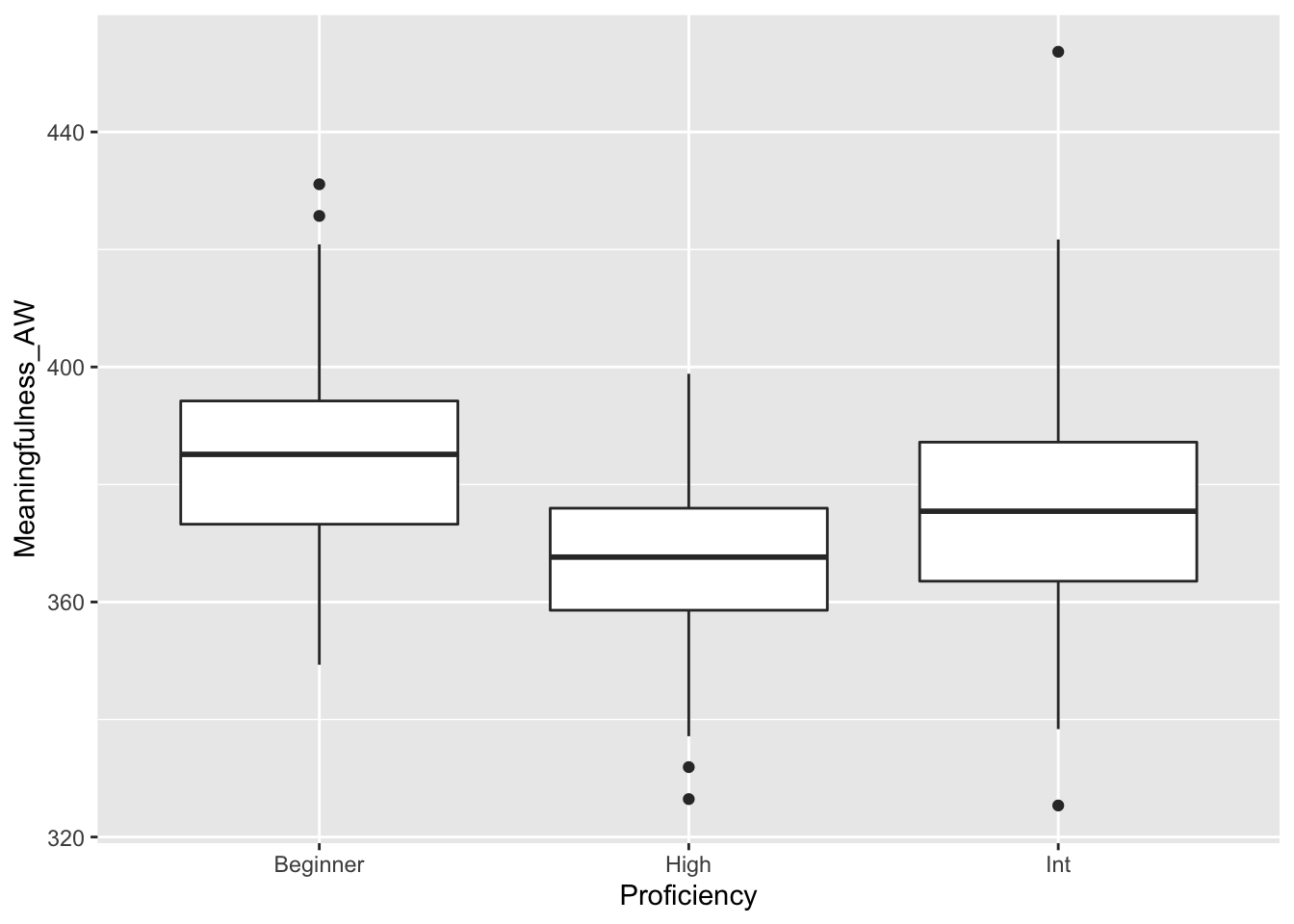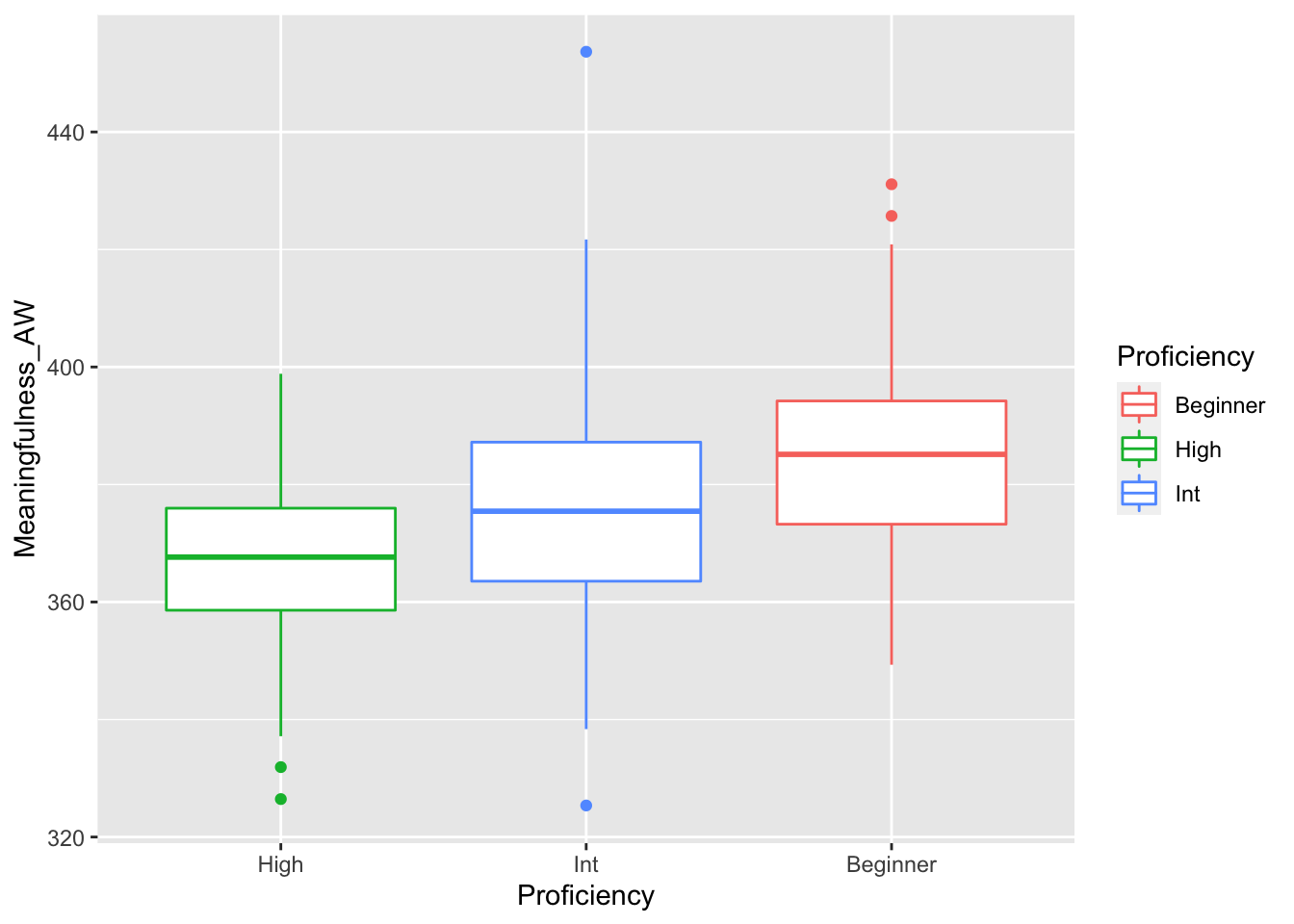## Examining differences between more than two groups

When we want to examine differences between two independent groups, a t-test or Man-Whitney U test are the most appropriate to use. If we have more than two independent groups, however, it is not appropriate to use these tests. Instead, we should use an Analysis of Variance (ANOVA) test or one of the non-parametric alternatives.

### Assumptions of One-Way ANOVA

To use an ANOVA, our data must meet the following assumptions:

• The groups are independent (and do not include repeated measures)
• There is a normal distribution of values in each group
• The variance in each group is equal (this is call homogeneity of variance)
• The data is continuous

### Description of sample data

In this tutorial, we will be looking at essays written by individuals at three different levels of lexical proficiency (Beginner, Intermediate, and High). We will also be looking at the average meaningfulness score for all words in each text. Meaningfulness scores are one way of determining how many concepts a particular word might be related to (e.g., the word “food” would be more “meaningful” than “zygote” because it could be used in more semantic contexts). We will be determining whether there are any differences in average meaningfulness scores based on lexical proficiency group.

mydata <- read.csv("data/anova_sample.csv", header = TRUE)
summary(mydata)
##    filenames     Proficiency  Frequent_Trigram_Proportion
##  1.txt  :  1   Beginner: 61   Min.   :0.0480
##  10.txt :  1   High    : 68   1st Qu.:0.1390
##  100.txt:  1   Int     :111   Median :0.1931
##  101.txt:  1                  Mean   :0.1930
##  102.txt:  1                  3rd Qu.:0.2427
##  103.txt:  1                  Max.   :0.3623
##  (Other):234
##  Meaningfulness_AW Register_Range_CW L1_Word_Reaction_Time Concreteness_AW
##  Min.   :325.4     Min.   :10.23     Min.   :594.3         Min.   :2.143
##  1st Qu.:363.7     1st Qu.:13.13     1st Qu.:610.5         1st Qu.:2.491
##  Median :375.4     Median :13.57     Median :615.9         Median :2.619
##  Mean   :376.1     Mean   :13.52     Mean   :616.4         Mean   :2.644
##  3rd Qu.:386.3     3rd Qu.:13.93     3rd Qu.:621.4         3rd Qu.:2.772
##  Max.   :453.7     Max.   :14.86     Max.   :655.6         Max.   :3.539
## 

### Checking assumptions, visualizing the data

#### Checking the assumption of normality

First, we can create density plots to look at the distribution of each group. These density plots suggest that the data is roughly normal and that the variance is roughly equal.

library(ggplot2)
ggplot(mydata, aes(x=Meaningfulness_AW, color = Proficiency, fill=Proficiency)) +
geom_density(alpha = 0.4) #note that "alpha" sets the level of color transparencyWe can also run a Shapiro-Wilk test for each group to test for normality. To do so, we create a dataframe for each proficiency group using dplyr(), then run the Shapiro-Wilk test for each group.

#load dplyr package, which helps us manipulate datasets:
library(dplyr)

#create a new dataframe that includes only Beginner:
beginner.ds <- mydata %>% filter(Proficiency == "Beginner")
#create a new dataframe that includes only Int:
intermediate.ds <- mydata %>% filter(Proficiency == "Int")
#create a new dataframe that includes only High:
high.ds <- mydata %>% filter(Proficiency == "High")

#Test normality for Meaningfulness_AW in Beginner essays
shapiro.test(beginner.ds$Meaningfulness_AW) #p = 0.2336 ## ## Shapiro-Wilk normality test ## ## data: beginner.ds$Meaningfulness_AW
## W = 0.97457, p-value = 0.2336
#Test normality for Meaningfulness_AW in Int essays
shapiro.test(intermediate.ds$Meaningfulness_AW) #p = 0.0112 ## ## Shapiro-Wilk normality test ## ## data: intermediate.ds$Meaningfulness_AW
## W = 0.96912, p-value = 0.0112
#Test normality for Meaningfulness_AW in High essays
shapiro.test(high.ds$Meaningfulness_AW) #p = 0.1591 ## ## Shapiro-Wilk normality test ## ## data: high.ds$Meaningfulness_AW
## W = 0.97366, p-value = 0.1591

The results indicate that Meaningfulness_AW scores Beginner and High essays do not violate the assumption of normality, while Meaningfulness_AW scores for Int essays do (p = 0.011). As mentioned in previous tutorials, the Shapiro-Wilk test is rather stringent, so we can decide whether we think it is appropriate to use a parametric or non-parametric test based on the visual inspection and on the Shapiro-Wilk test results.

#### Checking the assumption of equal variance

Second, we will check the assumption of equal variance (also known as homogeneity of variance). First, we will generate some boxplots, which (along with the distribution plots above), help give an indication of whether our groups have roughly equal variance.

ggplot(data = mydata) +
geom_boxplot(mapping = aes(x = Proficiency, y = Meaningfulness_AW))As you likely noticed in the boxplot above, ggplot organizes groups alphabetically by default (the order above is Beginner, High, Intermediate).

However, we can adjust this using the reorder function (see below):

ggplot(data = mydata) +
geom_boxplot(aes(x = reorder(Proficiency, Meaningfulness_AW, FUN = median), y = Meaningfulness_AW, color = Proficiency)) +
xlab("Proficiency")These boxplots support the notion that the variance is roughly equal, and also suggests that there may be meaningful differences between the groups.

We can also use Levene’s test to statistically assess whether the variance is roughly equal between groups.

library(car)
leveneTest(Meaningfulness_AW ~ Proficiency, mydata) #the syntax here is variable ~ grouping variable, dataframe
## Levene's Test for Homogeneity of Variance (center = median)
##        Df F value Pr(>F)
## group   2  2.0676 0.1288
##       237

The results of Levene’s test indicate that the variance is roughly equal across our three proficiency groups, which means that our data meets this assumption.

### Conducting a one-way Analysis of Variance (ANOVA)

First, lets revisit the assumptions of an ANOVA:

• The groups are independent (this assumption is met as each essay was written by a different individual)
• There is a normal distribution of values in each group (this is arguably accurate based on visual inspection, though the intermediate group is not normally distributed based on the Shapiro-Wilk test)
• The variance in each group is equal (visual inspection of boxplots and Levene’s test indicate that this assumption is met)
• The data is continuous (this assumption is met. Meaningfulness_AW is continuous)

Now, lets run a one-way ANOVA to determine whether there are any differences between our groups. Note that an ANOVA will NOT tell us where the differences are (e.g., between which [or all] groups). An ANOVA only tells us whether there are any differences. To find out where differences exist (if any are found), we have to do post-hoc analyses.

To determine whether any differences exist, we create a model called “anova_model” using the aov() function. We then use the summary() function to get the model statistics.

library(lsr)
anova_model <- aov(Meaningfulness_AW~Proficiency, data = mydata)
summary(anova_model)
##              Df Sum Sq Mean Sq F value   Pr(>F)
## Proficiency   2  11377    5689   20.53 6.01e-09 ***
## Residuals   237  65682     277
## ---
## Signif. codes:  0 '***' 0.001 '**' 0.01 '*' 0.05 '.' 0.1 ' ' 1

There are a lot of numbers above, but for now we will only discuss Pr(>F) (this is our p value). As we can see, there is a significant difference between our groups (p < .05).

To determine how “big” the difference(s) are, we will use the etaSquared() function. Note that the rules of thumb for etaSquared are as follows:

• .100-.299 (small)
• .300-.599 (medium)
• .600 - 1.000 (large)
etaSquared(anova_model, type = 3)
##               eta.sq eta.sq.part
## Proficiency 0.147645    0.147645

As we see from the results, our effect is rather small (eta_squared = 0.148).

#### Conducting pairwise comparisons

To figure out where the differences are, we have to run pairwise comparisons. In this case, we will conduct Tukey HSD (Honest Significant Differences) test, which nicely balances the potential for Type I and Type II errors. As we see below, there are significant differences between each of our groups (look at the p adj column, which reports a p value that is adjusted for the number of comparisons made).

TukeyHSD(anova_model)
##   Tukey multiple comparisons of means
##     95% family-wise confidence level
##
## Fit: aov(formula = Meaningfulness_AW ~ Proficiency, data = mydata)
##
## $Proficiency ## diff lwr upr p adj ## High-Beginner -18.801696 -25.72585 -11.877543 0.0000000 ## Int-Beginner -9.491098 -15.74900 -3.233192 0.0012211 ## Int-High 9.310598 3.26413 15.357066 0.0010025 To get an effect size for each contrast, we will have to do a little more work. Below, we create a new dataframe using the “filter” and “select” functions of dplyr to select only rows that have a Proficiency value of “Beginner” or “Int” (using filter), and only the columns Proficiency and Meaningfulness_AW. We then use the function “cohen.d” in the psych package to calculate the effect size between the “Beginner” and “Int” groups. As we see below, the effect between these groups is .54, which represents a medium effect. #create new df with only Beginner and Int mydata_beg_int <- mydata %>% filter(Proficiency == "Beginner" | Proficiency == "Int") %>% select(Proficiency, Meaningfulness_AW) #summary(mydata_effs_BI) library(psych) cohen.d(mydata_beg_int,"Proficiency")# (-).54 [medium effect] ## Call: cohen.d(x = mydata_beg_int, group = "Proficiency") ## Cohen d statistic of difference between two means ## lower effect upper ## Meaningfulness_AW -0.87 -0.54 -0.21 ## ## Multivariate (Mahalanobis) distance between groups ##  0.54 ## r equivalent of difference between two means ## Meaningfulness_AW ## -0.25 To get the effect sizes for the rest of the contrasts, we repeat this process with the other group combinations (“Beginner” to “High” and “Int” to “High”). As we see below, there is a large effect for the difference between Beginner and High (d = 1.2), and a medium effect for the difference between Int and High (d = ) #create new df with only Beginner and High mydata_beg_high <- mydata %>% filter(Proficiency == "Beginner" | Proficiency == "High") %>% select(Proficiency, Meaningfulness_AW) #summary(mydata_effs_BH) #create new df with only Int and High mydata_int_high <- mydata %>% filter(Proficiency == "Int" | Proficiency == "High") %>% select(Proficiency, Meaningfulness_AW) #summary(mydata_effs_IH) cohen.d(mydata_beg_high,"Proficiency")# (-)1.2 [large effect] ## Call: cohen.d(x = mydata_beg_high, group = "Proficiency") ## Cohen d statistic of difference between two means ## lower effect upper ## Meaningfulness_AW -1.7 -1.2 -0.83 ## ## Multivariate (Mahalanobis) distance between groups ##  1.2 ## r equivalent of difference between two means ## Meaningfulness_AW ## -0.53 cohen.d(mydata_int_high,"Proficiency")# .56 [medium effect] ## Call: cohen.d(x = mydata_int_high, group = "Proficiency") ## Cohen d statistic of difference between two means ## lower effect upper ## Meaningfulness_AW 0.24 0.56 0.88 ## ## Multivariate (Mahalanobis) distance between groups ##  0.56 ## r equivalent of difference between two means ## Meaningfulness_AW ## 0.26 ### How to compare groups if we do not meet the assumptions of a one-way ANOVA? #### What to do if we violate homogeneity of variance If our groups are normally distributed but violate homogeneity of variance, we can compute the Welch one-way test with Games-Howell post hoc tests. Note that we would still use eta squared as our effect size, which would would generate using the code above. welch_model <- oneway.test(Meaningfulness_AW~Proficiency, data = mydata,var.equal = FALSE) print(welch_model) ## ## One-way analysis of means (not assuming equal variances) ## ## data: Meaningfulness_AW and Proficiency ## F = 24.494, num df = 2.00, denom df = 142.05, p-value = 7.255e-10 library(userfriendlyscience) posthocTGH(mydata$Proficiency, y = mydata\$Meaningfulness_AW)
##            n means variances
## Beginner  61   386       278
## High      68   367       189
## Int      111   376       330
##
##                diff ci.lo ci.hi   t  df    p
## High-Beginner -18.8 -25.2   -12 6.9 117 <.01
## Int-Beginner   -9.5 -16.0    -3 3.5 133 <.01
## Int-High        9.3   3.6    15 3.9 169 <.01

#### What if my data isn’t normally distributed?

If our data is NOT normally distributed, there are non-parametric alternatives. One of these is the Kruskal-Wallis rank sum test:

kruskal.test(Meaningfulness_AW~Proficiency, data = mydata)
##
##  Kruskal-Wallis rank sum test
##
## data:  Meaningfulness_AW by Proficiency
## Kruskal-Wallis chi-squared = 36.999, df = 2, p-value = 9.244e-09

One option for pairwise comparisons with the Kruskal- Wallis test is the Dunn test:

library(FSA)
dunnTest(Meaningfulness_AW~Proficiency, data = mydata, method = "bh")
##        Comparison         Z      P.unadj        P.adj
## 1 Beginner - High  6.077392 1.221526e-09 3.664578e-09
## 2  Beginner - Int  3.339410 8.395669e-04 8.395669e-04
## 3      High - Int -3.503382 4.593903e-04 6.890855e-04